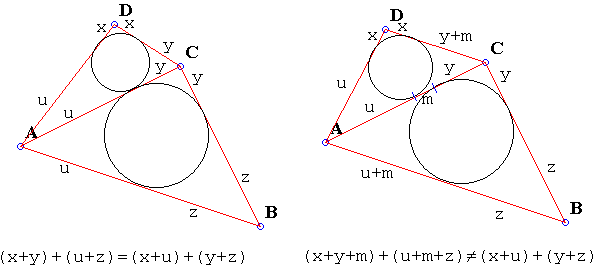# Pairs of Incircles in a Quadrilateral What is it about? A Mathematical Droodle

Explanation### Explanation

Together with its sides, the diagonals of a quadrilateral form two pairs of triangles: ABC, ADC and BCD, BAD. One pair shares the side AC, the other the side BD. In each of the four triangles draw its incircle. The applet illustrates the following theorem: the two incircles in one pair touch each other iff the same is true of the two incircles in the other pair. (Two incircles of adjacent triangles may only touch each other if the points where they touch the common side coincide.)The theorem follows from the easily verifiable fact that both conditions are equivalent to the quadrilateral having the property AB + CD = BC + AD. The latter is characterizatic of inscriptable quadrilaterals. (That AB + CD = BC + AD holds for inscriptable quadrilaterals is obvious. The proof in the opposite direction is by contradiction.)

If we allow the quadrilateral to degenerate into a triangle, the result just obtained leads to a nice theorem in a triangle: Let the incircle of ΔABC touch the side BC at point X. Then incircles of triangles ABX and ACX are tangent on line AX.